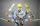Two valves

Water fill the pool by two valves for 11 days. After 7 days the first valve was stopped and the second valve fill pool for 7 days.

How many days took to fill the pool each of the valves individually?

Result

x =  25.67 d
y =  19.25 d

Solution:Leave us a comment of this math problem and its solution (i.e. if it is still somewhat unclear...):Be the first to comment!To solve this verbal math problem are needed these knowledge from mathematics:

Looking for help with calculating harmonic mean? Looking for a statistical calculator? Do you have a system of equations and looking for calculator system of linear equations?

Next similar math problems:

1. Hectoliters of waterThe pool has a total of 126 hectoliters of water. The first pump draws 2.1 liters of water per second. A second pump pumps 3.5 liters of water per second. How long will it take both pumps to drain four-fifths of the water at the same time?
2. BricklayersWhen a bricklayer works himself to redeem the house in 8 days, the other bricklayer will be finished in 10 days. How long will it take to make 3 such houses together?
3. Father 7Father is 6 times older than his son. After 4 years, the father will only be 4 times older. What are their present ages?
4. Two numbersWe have two numbers. Their sum is 140. One-fifth of the first number is equal to half the second number. Determine those unknown numbers.
5. Minute averageIn a factory, four workers are assigned to complete an order received for dispatching 1400 boxes of a particular commodity. Worker A takes 4 mins per box, Worker B takes 6 minutes per box, C takes 10 mins per box, D takes 15 mins per box. Find the average.
6. Two equationsSolve equations (use adding and subtracting of linear equations): -4x+11y=5 6x-11y=-5
7. Theorem proveWe want to prove the sentence: If the natural number n is divisible by six, then n is divisible by three. From what assumption we started?
8. Jane plantsJane plants flowers in the garden. If she planted 12 every hour instead of 9 flowers, she would finish with the job an hour earlier. How many flowers does she plant?
9. Elimination methodSolve system of linear equations by elimination method: 5/2x + 3/5y= 4/15 1/2x + 2/5y= 2/15
10. TheatroTheatrical performance was attended by 480 spectators. Women were in the audience 40 more than men and children 60 less than half of adult spectators. How many men, women and children attended a theater performance?
11. EquationsSolve following system of equations: 6(x+7)+4(y-5)=12 2(x+y)-3(-2x+4y)=-44
12. Three unknownsSolve the system of linear equations with three unknowns: A + B + C = 14 B - A - C = 4 2A - B + C = 0
13. Lee isLee is 8 years more than twice Park's age, 4 years ago, Lee was three times as old. How old was Lee 4 years ago?
14. Three brothersThe three brothers have a total of 42 years. Jan is five years younger than Peter and Peter is 2 years younger than Michael. How many years has each of them?
15. FamilyFamily has 4 children. Ondra is 3 years older than Matthew and Karlos 5 years older than the youngest Jane. We know that they are together 30 years and 3 years ago they were together 19 years. Determine how old the children are.
16. Linear systemSolve this linear system (two linear equations with two unknowns): x+y =36 19x+22y=720
17. Hotel roomsIn the 45 rooms, there were 169 guests, some rooms were three-bedrooms and some five-bedrooms. How many rooms were?